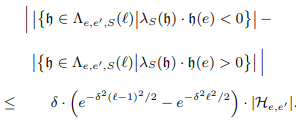# Distributed Computation of the Mode

ContentAbstract

This paper studies the problem of computing the most frequent element (the mode) by means of a distributed algorithm where the elements are located at the nodes of a network. Let k denote the number of distinct elements and further let mi be the number of occurrences of the element ei in the ordered list of occurrences m1 > m2 ≥ ... ≥ mk. We give a deterministic distributed algorithm with time complexity O(D+k) where D denotes the diameter of the graph, which is essentially tight. As our main contribution, a Monte Carlo algorithm is presented which computes the mode in O(D + F2/m2 1 · log k) time with high probability, where the frequency moment F` is de�ned as F` = Pk i=1 m` i . This algorithm is substantially faster than the deterministic algorithm for various relevant frequency distributions. Moreover, we provide a lower bound of Ω(D +F5/(m5 1B)), where B is the maximum message size, that captures the e�ect of the frequency distribution on the time complexity to compute the mode.Top
Authors
• Locher, Thomas
• Kuhn, Fabian
• Schmid, StefanTop
Supplemental Material
Shortfacts
 Category Paper in Conference Proceedings or in Workshop Proceedings (Paper) Event Title 27th Annual ACM Symposium on Principles of Distributed Computing (PODC) Divisions Subjects Event Location Toronto, Canada Event Type Conference Event Dates August 2008 Date 2008 Export BibTeX ASCII Citation DIDL Dublin Core EP3 XML EP3 XML with files embedded EndNote Eprints Application Profile HTML Citation JSON METS MODS Multiline CSV OAI-ORE Resource Map (Atom Format) OAI-ORE Resource Map (RDF Format) OpenURL ContextObject RDF+N-Triples RDF+N3 RDF+XML Refer Reference Manager Simple MetadataTop